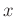# Field Example

To evaluate the performance of the proposed method in field conditions, we used a historic marine dataset from the Gulf of Mexico (Claerbout, 2000). Figure 7a shows the input data, in which near-offset information is completely missing. We removed 40% of randomly selected traces (Figure 7b) from the input data (Figure 7a). The velocity scanning from the missing data can still generate accurate parameter fields using equation 4. Figure 8a and Figure 8b show the velocity spectra andspectra, respectively. Equation 5 converts RMS velocity andto seismic pattern (Figure 8c), which displays the varying slopes in CMP gathers and common-offset sections. The generalized velocity-dependent (VD)-seislet transform compresses predictable information according to the local slopes along the offsets. We applied the modified Bregman iteration with the generalized VD-seislet transform to recover missing data. The results show that the missing traces were interpolated well even when anisotropy is present (Figure 9b). For comparison, we applied 3D Fourier transform with POCS (Figure 9a). The Fourier transform could not recover the missing information as accurately as the generalized VD-seislet transform. The results of the 3D Fourier POCS method show obvious gaps caused by inaccurate interpolation, whereas the generalized VD-seislet and the modified Bregman iteration strategy provides a reasonably accurate result for interpolating missing data.

If we extend the generalized velocity-dependent (VD)-seislet transform domain along the transform scale axis and recalculate the slope field according to the new-coordinate axis in equation 5, the inverse generalized VD-seislet transform will accomplish trace interpolation of the input CMP gather (Figure 10a), which is selected from Figure 7a at the midpoint location 11.8925 km. We extended the generalized VD-seislet coefficients with small random noise to consider the existence of realistic noise on different scale levels. Figure 10b shows that the interpolated result provides four times more traces and removes most aliasing. Thus, trace interpolation is a natural operation in the generalized VD-seislet domain.bei,bei-zero
Figure 7.
Marine data with near-offset missing (a) and data with 40% traces removed (b).bei-vel,bei-s,bei-dip
Figure 8.
Velocity section (a) andsection (b) obtained by using equation 4, and velocity-dependent (VD) slopes (c).bfour3pocs,bei-seis2
Figure 9.
Interpolated results using different methods. 3D Fourier POCS method (a) and the proposed method (b).cmp1,cmp2
Figure 10.
CMP gather before (a) and after (b) trace interpolation. The interpolated gather has four times more traces than the original one shown in Figure 10a.2019-05-06